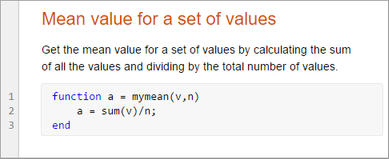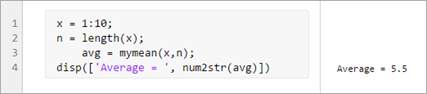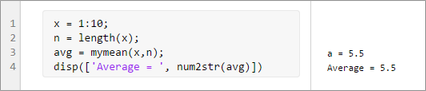## 创建实时函数

### 添加代码

```function a = mymean(v,n) a = sum(v)/n; end```

### 添加帮助### 运行实时函数

`mymean(1:10, 10)`
```ans = 5.5000```

```x = 1:10; n = length(x); avg = mymean(x,n); disp(['Average = ', num2str(avg)])``````function a = mymean(v,n) a = sum(v)/n; disp(['a = ', num2str(a)]) end```MATLAB Online 中，您可以使用按钮以交互方式运行实时函数。当您使用按钮运行实时函数时，输出会显示在命令行窗口中。要运行需要输入参数值或任何其他附加设置的实时函数，请通过点击并添加一个或多个命令来配置按钮。有关配置按钮的详细信息，请参阅为函数配置运行按钮

### 将实时函数另存为纯代码

1. 实时编辑器选项卡上的文件部分中，选择保存 > 另存为...

2. 在显示的对话框中，选择 MATLAB 代码文件 (UTF-8) (*.m) 作为保存类型

3. 点击